# Selina Solutions Concise Maths Class 10 Chapter 1 GST [Goods and Services Tax] Exercise 1(A)

GST is an indirect tax which is paid by consumers or organisations when they buy goods or services or both. In this exercise, students will be solving problems on the calculation of GST. The solutions for all these problems are prepared in a comprehensive manner and are available in Selina Solutions for Class 10 Maths. As we know that Class 10 is a major part for students to achieve their future goals. Students who want more knowledge about the concepts which are discussed in this exercise can download Concise Selina Solutions for Class 10 Maths Chapter 1 GST [Goods and Services Tax] Exercise 1(A) PDF provided below.

## Selina Solutions Concise Maths Class 10 Chapter 1 GST [Goods and Services Tax] Exercise 1(A) Download PDF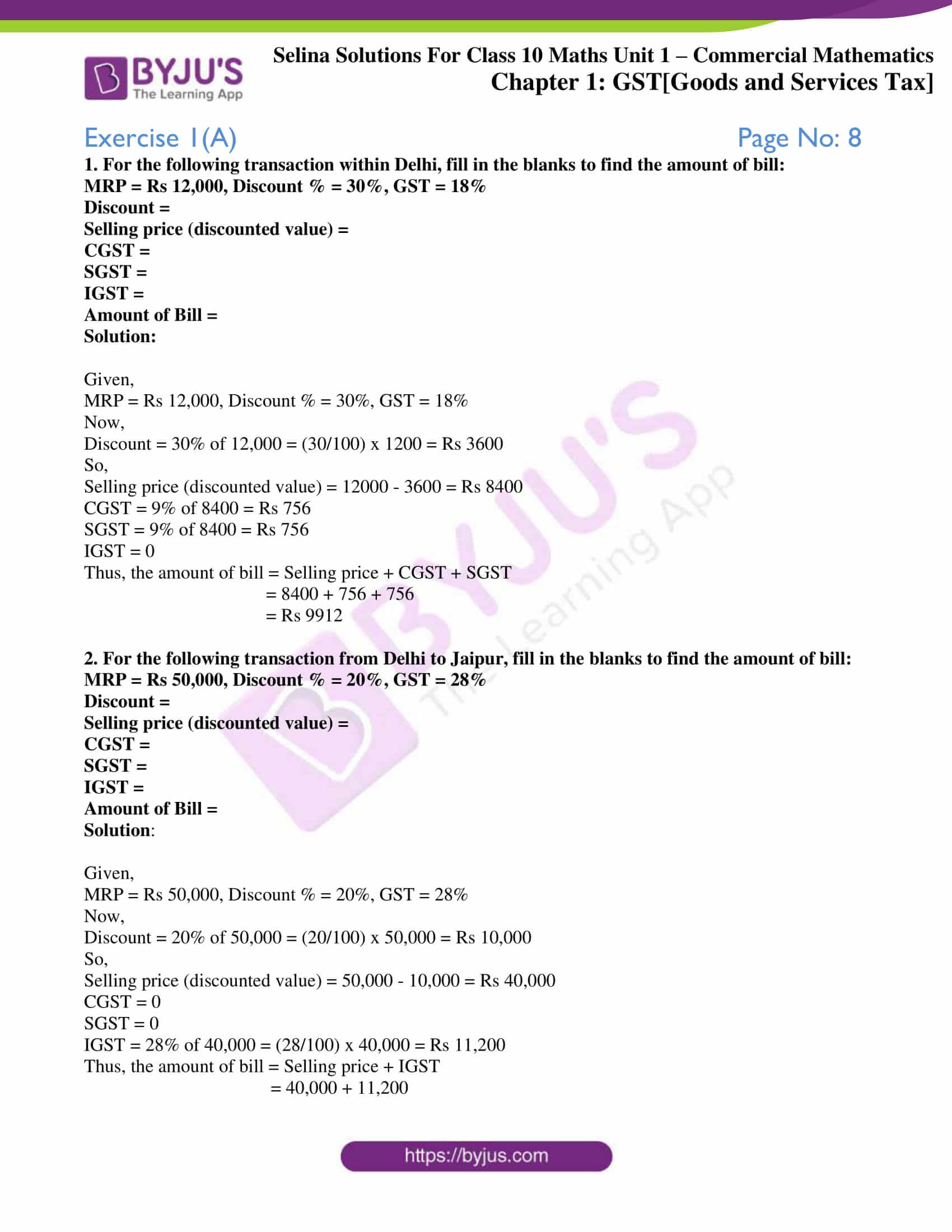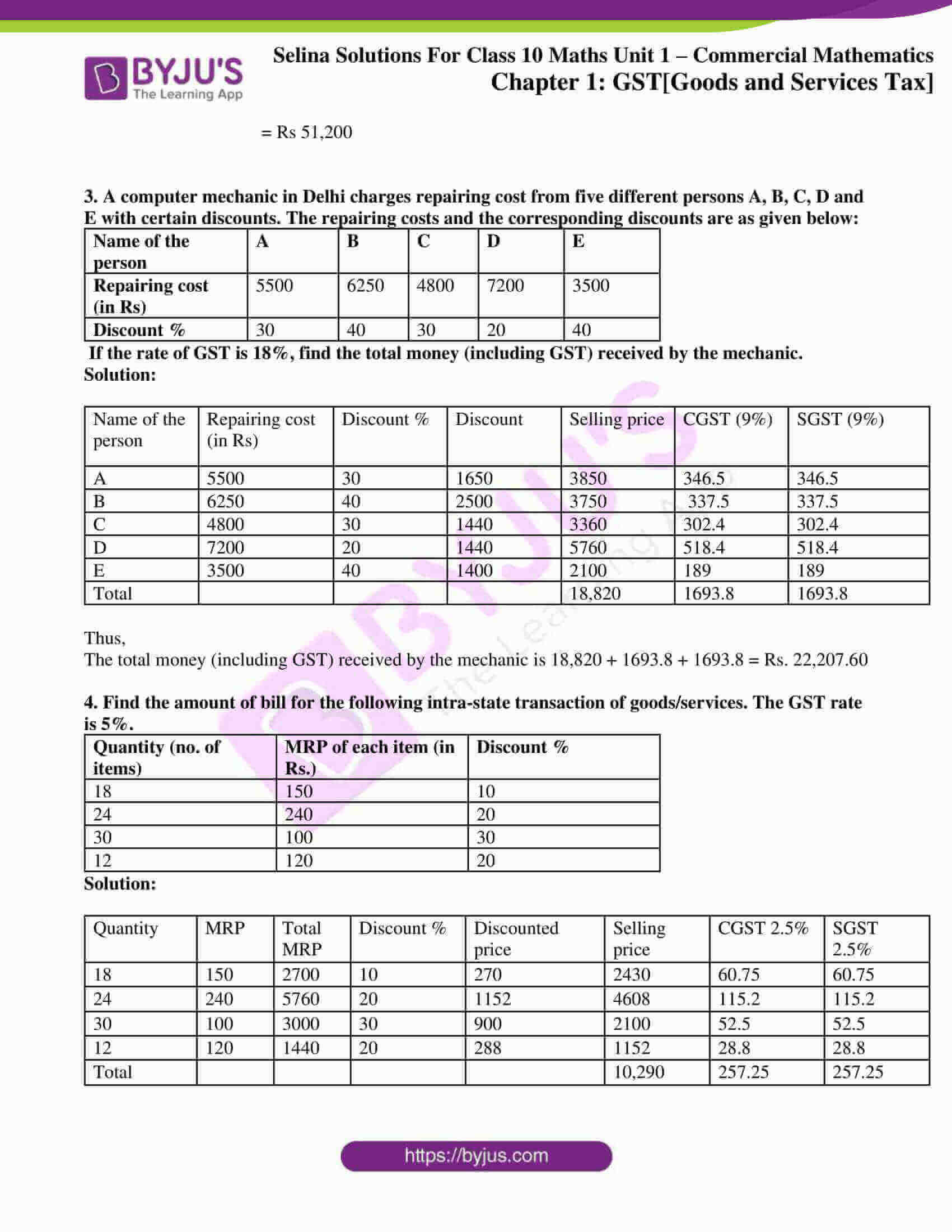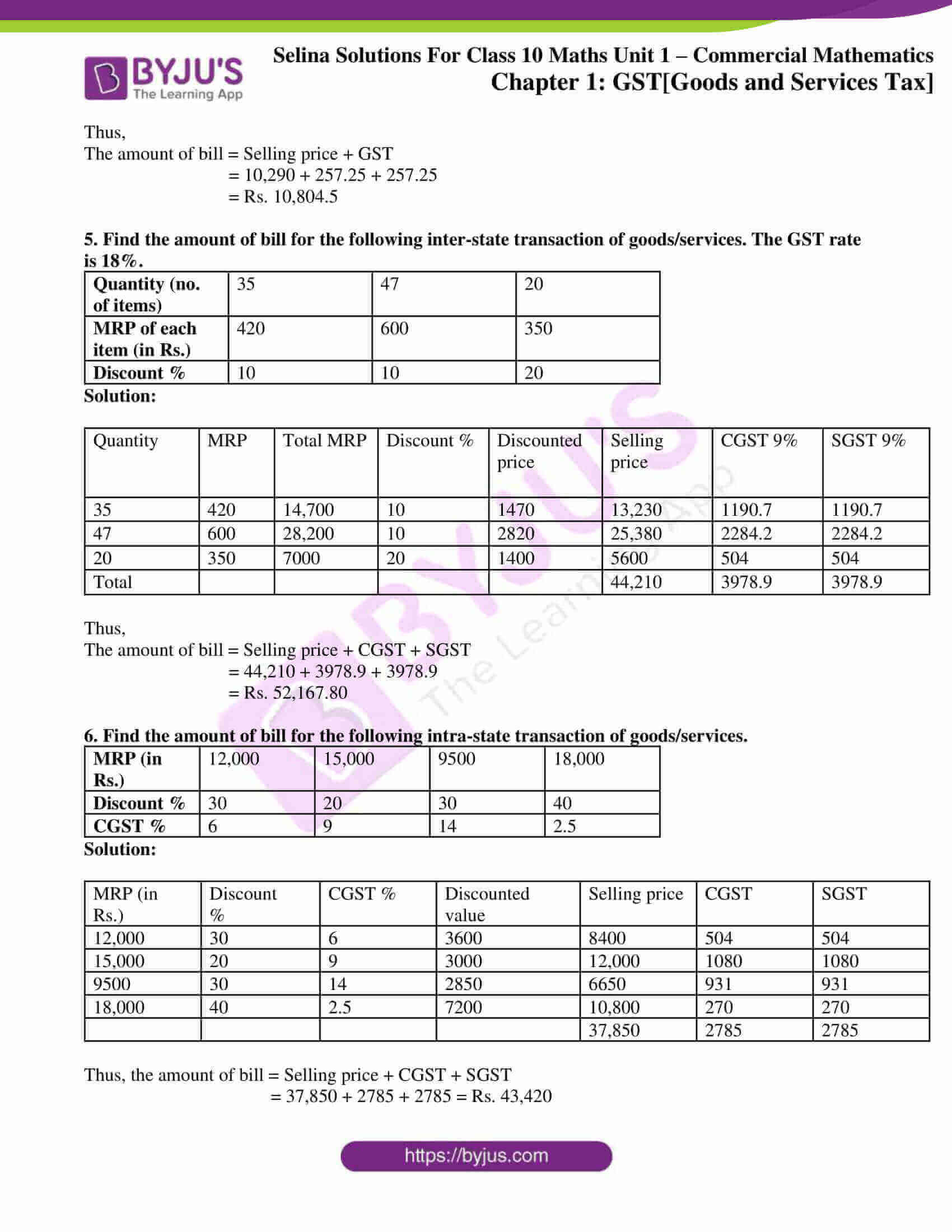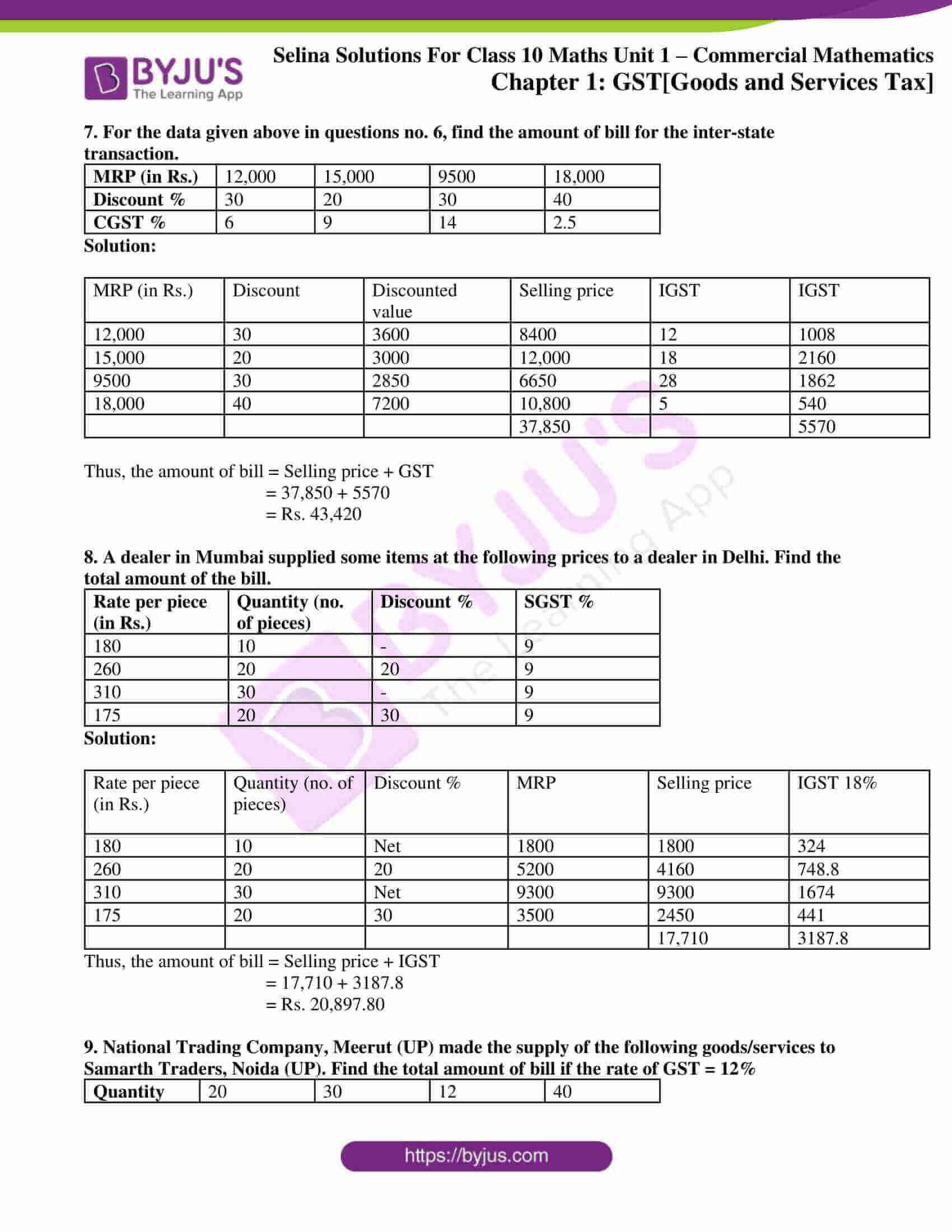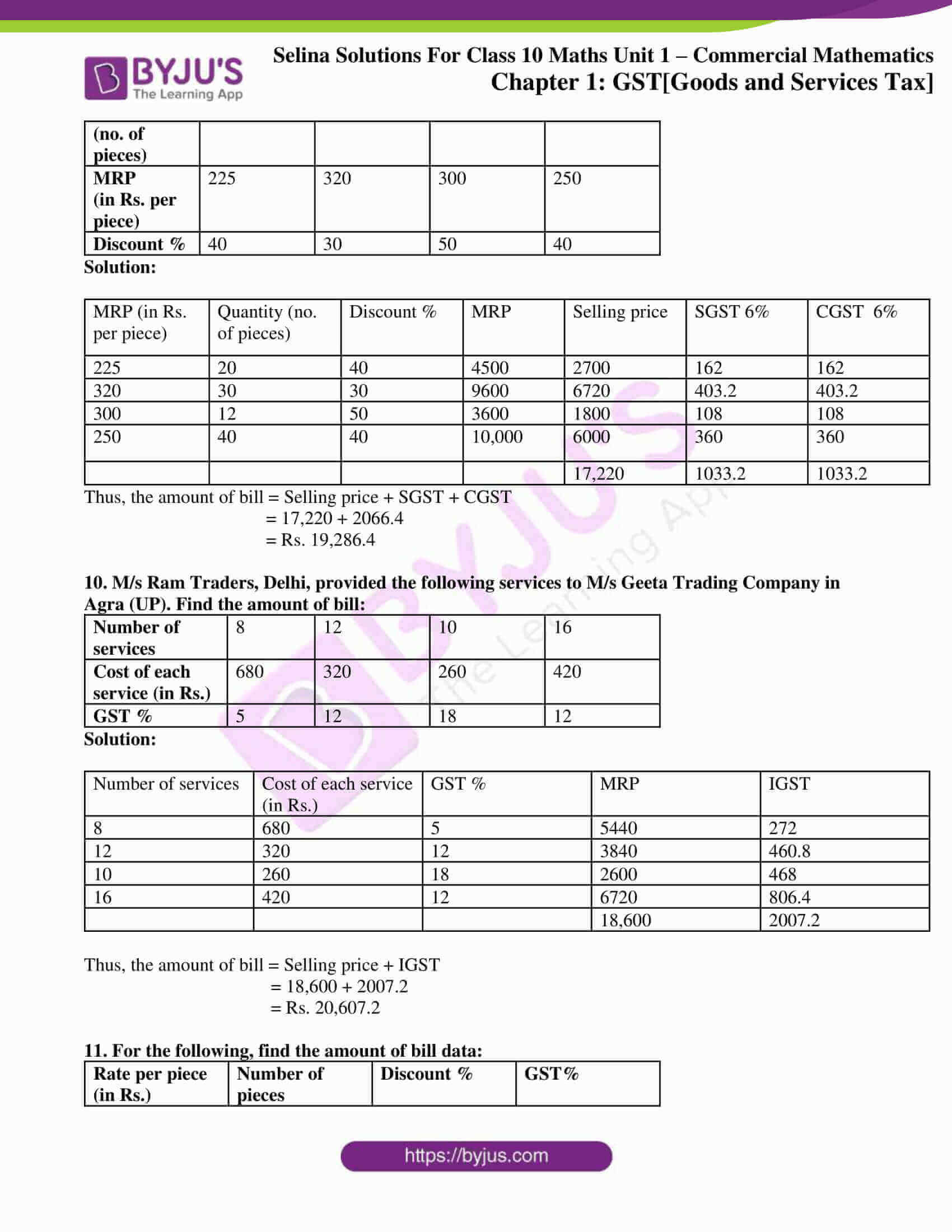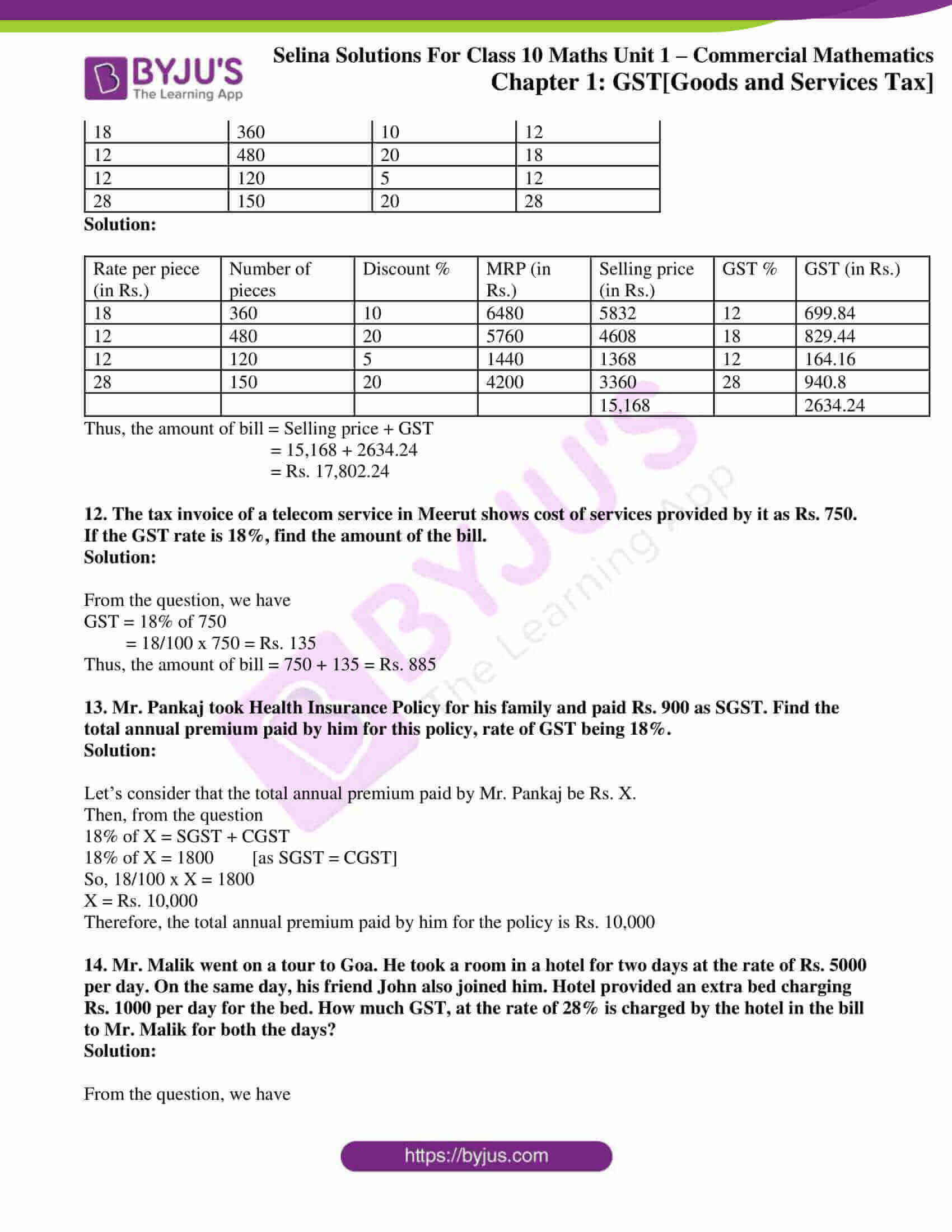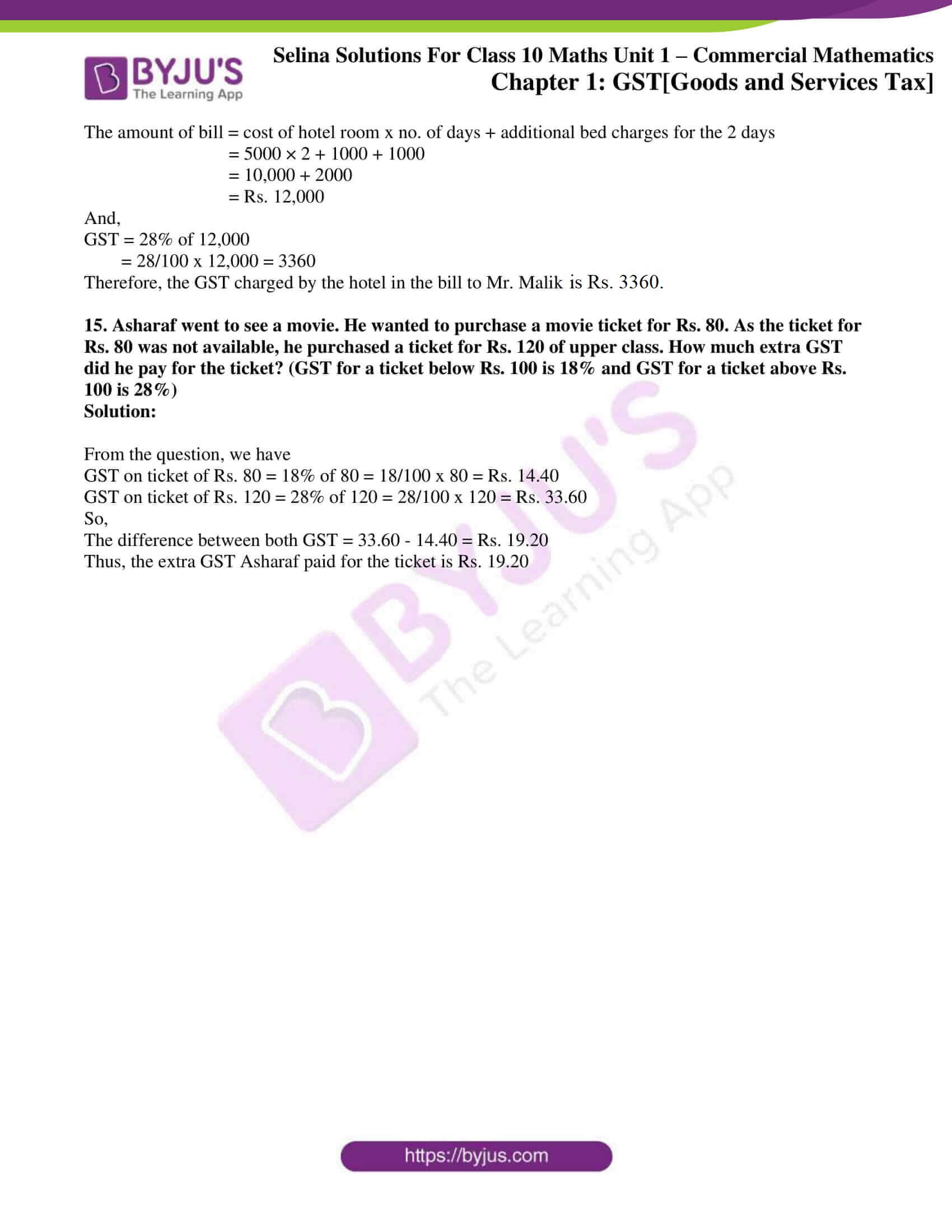### Access another exercise of Selina Solutions Concise Maths Class 10 Chapter 1 GST [Goods and Services Tax]

Exercise 1(B) Solutions

### Exercise 1(A) Page No: 8

1. For the following transaction within Delhi, fill in the blanks to find the amount of bill:

MRP = Rs 12,000, Discount % = 30%, GST = 18%

Discount =

Selling price (discounted value) =

CGST =

SGST =

IGST =

Amount of Bill =

Solution:

Given,

MRP = Rs 12,000, Discount % = 30%, GST = 18%

Now,

Discount = 30% of 12,000 = (30/100) x 1200 = Rs 3600

So,

Selling price (discounted value) = 12000 – 3600 = Rs 8400

CGST = 9% of 8400 = Rs 756

SGST = 9% of 8400 = Rs 756

IGST = 0

Thus, the amount of bill = Selling price + CGST + SGST

= 8400 + 756 + 756

= Rs 9912

2. For the following transaction from Delhi to Jaipur, fill in the blanks to find the amount of bill:

MRP = Rs 50,000, Discount % = 20%, GST = 28%

Discount =

Selling price (discounted value) =

CGST =

SGST =

IGST =

Amount of Bill =

Solution:

Given,

MRP = Rs 50,000, Discount % = 20%, GST = 28%

Now,

Discount = 20% of 50,000 = (20/100) x 50,000 = Rs 10,000

So,

Selling price (discounted value) = 50,000 – 10,000 = Rs 40,000

CGST = 0

SGST = 0

IGST = 28% of 40,000 = (28/100) x 40,000 = Rs 11,200

Thus, the amount of bill = Selling price + IGST

= 40,000 + 11,200

= Rs 51,200

3. A computer mechanic in Delhi charges repairing cost from five different persons A, B, C, D and E with certain discounts. The repairing costs and the corresponding discounts are as given below:

 Name of the person A B C D E Repairing cost (in Rs) 5500 6250 4800 7200 3500 Discount % 30 40 30 20 40

If the rate of GST is 18%, find the total money (including GST) received by the mechanic.

Solution:

 Name of the person Repairing cost (in Rs) Discount % Discount Selling price CGST (9%) SGST (9%) A 5500 30 1650 3850 346.5 346.5 B 6250 40 2500 3750 337.5 337.5 C 4800 30 1440 3360 302.4 302.4 D 7200 20 1440 5760 518.4 518.4 E 3500 40 1400 2100 189 189 Total 18,820 1693.8 1693.8

Thus,

The total money (including GST) received by the mechanic is 18,820 + 1693.8 + 1693.8 = Rs. 22,207.60

4. Find the amount of bill for the following intra-state transaction of goods/services. The GST rate is 5%.

 Quantity (no. of items) MRP of each item (in Rs.) Discount % 18 150 10 24 240 20 30 100 30 12 120 20

Solution:

 Quantity MRP Total MRP Discount % Discounted price Selling price CGST 2.5% SGST 2.5% 18 150 2700 10 270 2430 60.75 60.75 24 240 5760 20 1152 4608 115.2 115.2 30 100 3000 30 900 2100 52.5 52.5 12 120 1440 20 288 1152 28.8 28.8 Total 10,290 257.25 257.25

Thus,

The amount of bill = Selling price + GST

= 10,290 + 257.25 + 257.25

= Rs. 10,804.5

5. Find the amount of bill for the following inter-state transaction of goods/services. The GST rate is 18%.

 Quantity (no. of items) 35 47 20 MRP of each item (in Rs.) 420 600 350 Discount % 10 10 20

Solution:

 Quantity MRP Total MRP Discount % Discounted price Selling price CGST 9% SGST 9% 35 420 14,700 10 1470 13,230 1190.7 1190.7 47 600 28,200 10 2820 25,380 2284.2 2284.2 20 350 7000 20 1400 5600 504 504 Total 44,210 3978.9 3978.9

Thus,

The amount of bill = Selling price + CGST + SGST

= 44,210 + 3978.9 + 3978.9

= Rs. 52,167.80

6. Find the amount of bill for the following intra-state transaction of goods/services.

 MRP (in Rs.) 12,000 15,000 9500 18,000 Discount % 30 20 30 40 CGST % 6 9 14 2.5

Solution:

 MRP (in Rs.) Discount % CGST % Discounted value Selling price CGST SGST 12,000 30 6 3600 8400 504 504 15,000 20 9 3000 12,000 1080 1080 9500 30 14 2850 6650 931 931 18,000 40 2.5 7200 10,800 270 270 37,850 2785 2785

Thus, the amount of bill = Selling price + CGST + SGST

= 37,850 + 2785 + 2785 = Rs. 43,420

7. For the data given above in questions no. 6, find the amount of bill for the inter-state transaction.

 MRP (in Rs.) 12,000 15,000 9500 18,000 Discount % 30 20 30 40 CGST % 6 9 14 2.5

Solution:

 MRP (in Rs.) Discount Discounted value Selling price IGST IGST 12,000 30 3600 8400 12 1008 15,000 20 3000 12,000 18 2160 9500 30 2850 6650 28 1862 18,000 40 7200 10,800 5 540 37,850 5570

Thus, the amount of bill = Selling price + GST

= 37,850 + 5570

= Rs. 43,420

8. A dealer in Mumbai supplied some items at the following prices to a dealer in Delhi. Find the total amount of the bill.

 Rate per piece (in Rs.) Quantity (no. of pieces) Discount % SGST % 180 10 – 9 260 20 20 9 310 30 – 9 175 20 30 9

Solution:

 Rate per piece (in Rs.) Quantity (no. of pieces) Discount % MRP Selling price IGST 18% 180 10 Net 1800 1800 324 260 20 20 5200 4160 748.8 310 30 Net 9300 9300 1674 175 20 30 3500 2450 441 17,710 3187.8

Thus, the amount of bill = Selling price + IGST

= 17,710 + 3187.8

= Rs. 20,897.80

9. National Trading Company, Meerut (UP) made the supply of the following goods/services to Samarth Traders, Noida (UP). Find the total amount of bill if the rate of GST = 12%

 Quantity (no. of pieces) 20 30 12 40 MRP (in Rs. per piece) 225 320 300 250 Discount % 40 30 50 40

Solution:

 MRP (in Rs. per piece) Quantity (no. of pieces) Discount % MRP Selling price SGST 6% CGST 6% 225 20 40 4500 2700 162 162 320 30 30 9600 6720 403.2 403.2 300 12 50 3600 1800 108 108 250 40 40 10,000 6000 360 360 17,220 1033.2 1033.2

Thus, the amount of bill = Selling price + SGST + CGST

= 17,220 + 2066.4

= Rs. 19,286.4

10. M/s Ram Traders, Delhi, provided the following services to M/s Geeta Trading Company in Agra (UP). Find the amount of bill:

 Number of services 8 12 10 16 Cost of each service (in Rs.) 680 320 260 420 GST % 5 12 18 12

Solution:

 Number of services Cost of each service (in Rs.) GST % MRP IGST 8 680 5 5440 272 12 320 12 3840 460.8 10 260 18 2600 468 16 420 12 6720 806.4 18,600 2007.2

Thus, the amount of bill = Selling price + IGST

= 18,600 + 2007.2

= Rs. 20,607.2

11. For the following, find the amount of bill data:

 Rate per piece (in Rs.) Number of pieces Discount % GST% 18 360 10 12 12 480 20 18 12 120 5 12 28 150 20 28

Solution:

 Rate per piece (in Rs.) Number of pieces Discount % MRP (in Rs.) Selling price (in Rs.) GST % GST (in Rs.) 18 360 10 6480 5832 12 699.84 12 480 20 5760 4608 18 829.44 12 120 5 1440 1368 12 164.16 28 150 20 4200 3360 28 940.8 15,168 2634.24

Thus, the amount of bill = Selling price + GST

= 15,168 + 2634.24

= Rs. 17,802.24

12. The tax invoice of a telecom service in Meerut shows cost of services provided by it as Rs. 750. If the GST rate is 18%, find the amount of the bill.

Solution:

From the question, we have

GST = 18% of 750

= 18/100 x 750 = Rs. 135

Thus, the amount of bill = 750 + 135 = Rs. 885

13. Mr. Pankaj took Health Insurance Policy for his family and paid Rs. 900 as SGST. Find the total annual premium paid by him for this policy, rate of GST being 18%.

Solution:

Let’s consider that the total annual premium paid by Mr. Pankaj be Rs. X.

Then, from the question

18% of X = SGST + CGST

18% of X = 1800  [as SGST = CGST]

So, 18/100 x X = 1800

X = Rs. 10,000

Therefore, the total annual premium paid by him for the policy is Rs. 10,000

14. Mr. Malik went on a tour to Goa. He took a room in a hotel for two days at the rate of Rs. 5000 per day. On the same day, his friend John also joined him. Hotel provided an extra bed charging Rs. 1000 per day for the bed. How much GST, at the rate of 28% is charged by the hotel in the bill to Mr. Malik for both the days?

Solution:

From the question, we have

The amount of bill = cost of hotel room x no. of days + additional bed charges for the 2 days

= 5000 × 2 + 1000 + 1000

= 10,000 + 2000

= Rs. 12,000

And,

GST = 28% of 12,000

= 28/100 x 12,000 = 3360

Therefore, the GST charged by the hotel in the bill to Mr. Malik is Rs. 3360.

15. Asharaf went to see a movie. He wanted to purchase a movie ticket for Rs. 80. As the ticket for Rs. 80 was not available, he purchased a ticket for Rs. 120 of upper class. How much extra GST did he pay for the ticket? (GST for a ticket below Rs. 100 is 18% and GST for a ticket above Rs. 100 is 28%)

Solution:

From the question, we have

GST on ticket of Rs. 80 = 18% of 80 = 18/100 x 80 = Rs. 14.40

GST on ticket of Rs. 120 = 28% of 120 = 28/100 x 120 = Rs. 33.60

So,

The difference between both GST = 33.60 – 14.40 = Rs. 19.20

Thus, the extra GST Asharaf paid for the ticket is Rs. 19.20